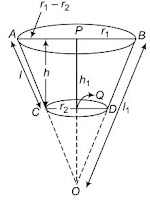# Class 10 Maths Chapter 13 Surface Areas and Volumes

NCERT Solutions for Class 10 Maths Chapter 13 Surface Areas and Volumes

Page No: 244

Exercise 13.1

Unless stated otherwise, take π = 22/7.

1. 2 cubes each of volume 64 cm3 are joined end to end. Find the surface area of the resulting cuboid.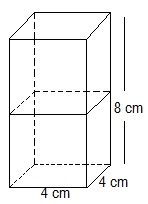Volume of each cube(a3) = 64 cm3
⇒ a3 = 64 cm3
⇒ a = 4 cm
Side of the cube = 4 cm
Length of the resulting cuboid = 4 cm
Breadth of the resulting cuboid = 4 cm
Height of the resulting cuboid = 8 cm
∴ Surface area of the cuboid = 2(lb + bh + lh)
= 2(8×4 + 4×4 + 4×8) cm2
= 2(32 + 16 + 32) cm2
= (2 × 80) cm2 = 160 cm2

2. A vessel is in the form of a hollow hemisphere mounted by a hollow cylinder. The diameter of the hemisphere is 14 cm and the total height of the vessel is 13 cm. Find the inner surface area of the vessel.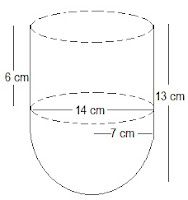Diameter of the hemisphere = 14 cm
Radius of the hemisphere(r) = 7 cm
Height of the cylinder(h) = 13 - 7 = 6 cm
Also, radius of the hollow hemisphere = 7 cm
Inner surface area of the vessel = CSA of the cylindrical part + CSA of hemispherical part
= (2πrh+2πr2) cm2 = 2πr(h+r) cm2
= 2 × 22/7 × 7 (6+7) cm2 = 572 cm2

3. A toy is in the form of a cone of radius 3.5 cm mounted on a hemisphere of same radius. The total height of the toy is 15.5 cm. Find the total surface area of the toy.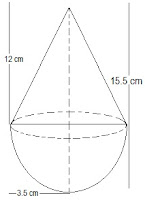Radius of the cone and the hemisphere(r) = 3.5 = 7/2 cm
Total height of the toy = 15.5 cm
Height of the cone(h) = 15.5 - 3.5 = 12 cmCurved Surface Area of cone = πrl = 22/7 × 7/2 × 25/2
= 275/2 cm2

Curved Surface Area of hemisphere = 2πr2
= 2 × 22/7 × (7/2)2
= 77 cm2

Total surface area of the toy = CSA of cone + CSA of hemisphere
= (275/2 + 77) cm2
= (275+154)/2 cm
= 429/2 cm2 = 214.5 cm2
The total surface area of the toy is 214.5 cm2

4. A cubical block of side 7 cm is surmounted by a hemisphere. What is the greatest diameter the hemisphere can have? Find the surface area of the solid.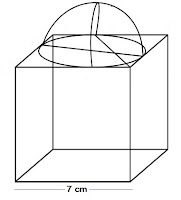Each side of cube = 7 cm
∴ Radius of the hemisphere = 7/2 cm
Total surface area of solid = Surface area of cubical block + CSA of hemisphere - Area of base of hemisphere
TSA of solid = 6×(side)+ 2πr- πr2
= 6×(side)+ πr2
= 6×(7)+ (22/7 × 7/2 × 7/2)
= (6×49) + (77/2)
= 294 + 38.5 = 332.5 cm2
The surface area of the solid is 332.5 cm2

5. A hemispherical depression is cut out from one face of a cubical wooden block such that the diameter l of the hemisphere is equal to the edge of the cube. Determine the surface area of the remaining solid.Diameter of hemisphere = Edge of cube = l
Total surface area of solid = Surface area of cube + CSA of hemisphere - Area of base of hemisphere
TSA of remaining solid = 6 (edge)+ 2πr- πr2
= 6l+ πr2
= 6l+ π(l/2)2
= 6l+ πl2/4
= l2/4 (24 + π) sq units

6. A medicine capsule is in the shape of a cylinder with two hemispheres stuck to each of its ends. The length of the entire capsule is 14 mm and the diameter of the capsule is 5 mm. Find its surface area.Two hemisphere and one cylinder are given in the figure.
Diameter of the capsule = 5 mm
∴ Radius = 5/2 = 2.5 mm
Length of the capsule = 14 mm
∴ Length of the cylinder = 14 - (2.5 + 2.5) = 9mm
Surface area of a hemisphere = 2πr2 = 2 × 22/7 × 2.5 × 2.5
= 275/7 mm2

Surface area of the cylinder = 2πrh
= 2 × 22/7 × 2.5 x 9
= 22/7 × 45
990/7 mm2

∴ Required surface area of medicine capsule
= 2 × Surface area of hemisphere + Surface area of cylinder
= (2 × 275/7) × 990/7
= 550/7 + 990/7
= 1540/7 = 220 mm2

Page No. 245

7. A tent is in the shape of a cylinder surmounted by a conical top. If the height and diameter of the cylindrical part are 2.1 m and 4 m respectively, and the slant height of the top is 2.8 m, find the area of the canvas used for making the tent. Also, find the cost of the canvas of the tent at the rate of Rs 500 per m2. (Note that the base of the tent will not be covered with canvas.)

Tent is combination of cylinder and cone.Diameter = 4 m
Slant height of the cone (l) = 2.8 m
Radius of the cone (r) = Radius of cylinder = 4/2 = 2 m
Height of the cylinder (h) = 2.1 m

∴ Required surface area of tent = Surface area of cone+Surface area of cylinder
= πrl + 2πrh
= πr(l+2h)
= 22/7 × 2 (2.8 + 2×2.1)
= 44/7 (2.8 + 4.2)
= 44/7 × 7 = 44 m2

Cost of the canvas of the tent at the rate of ₹500 per m2
= Surface area × cost per m2
= 44 × 500 = ₹22000

8. From a solid cylinder whose height is 2.4 cm and diameter 1.4 cm, a conical cavity of the
same height and same diameter is hollowed out. Find the total surface area of the
remaining solid to the nearest cm2.Diameter of cylinder = diameter of conical cavity = 1.4 cm
∴ Radius of cylinder = Radius of conical cavity = 1.4/2 = 0.7
Height of cylinder = Height of conical cavity = 2.4 cmTSA of remaining solid = Surface area of conical cavity+TSA of cylinder
= πrl + (2πrh + πr2)
= πr (l + 2h + r)
= 22/7 × 0.7 (2.5 + 4.8 + 0.7)
= 2.2 × 8 = 17.6 cm2

9. A wooden article was made by scooping out a hemisphere from each end of a solid cylinder, as shown in figure. If the height of the cylinder is 10 cm and its base is of radius 3.5 cm, find the total surface area of the article.Given,
Height of the cylinder, h = 10cm and radius of base of cylinder = Radius of hemisphere (r) = 3.5 cm
Now, required total surface area of the article = 2 × Surface area of hemisphere + Lateral surface area of cylinderExercise 13.2

1. A solid is in the shape of a cone standing on a hemisphere with both their radii being equal to 1 cm and the height of the cone is equal to its radius. Find the volume of the solid in terms of π.

Given, solid is a combination of a cone and a hemisphere.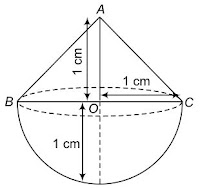Also, we have radius of the cone (r) = Radius of the hemisphere = 1cm and height of the cone (h) = 1cm
∴ Required volume of the solid = Volume of the cone + Volume of the hemisphere2. Rachel, an engineering, student was asked to make a model shaped like a cylinder with two cones attached at its two ends by using a thin aluminium sheet. The diameter of the model is 3 cm and its length is 12 cm. If each cone has a height of 2 cm, find the volume of air contained in the model that Rachel mode. (Assume the outer and inner dimensions of the model to be nearly the same.)

Given, model is a combination of a cylinder and two cones. Also, we have, diameter of the model,BC = ED = 3 cm.3. A gulab jamun, contains sugar syrup upto about 30% of its volume. Find approximately how much syrup would be found in 45 gulab jamuns, each shaped like a cylinder with two hemispherical ends with length 5 cm and diameter 2.8 cm (see figure).Let r be the radius of the hemisphere and cylinder both. h1 be the height of the hemisphere which is equal to its radius and h2 be the height of the cylinder.
Given, length = 5 cm, diameter = 2.8 cm4. A pen stand made of wood is in the shape of a cuboid with four conical depressions to hold pens. The dimensions of the cuboid are 15 cm by 10 cm by 3.5 cm. The radius of each of the depressions is 0.5 cm and the depth is 1.4 cm. Find the volume of wood in the entire stand (see figure).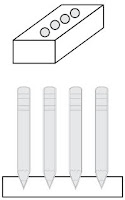Given, length of cuboid (l) = 15 cm
Breadth of cuboid (b) = 10cm
and height of cuboid (h) = 3.5 cm
∴ Volume of cuboid = l×b×h = 15 ×10 × 3.5 = 52535. A vessel is in the form of an inverted cone. Its height is 8 cm and the radius of its top, which is open, is 5 cm. It is filled with water upto the brim. When lead shots, each of which is a sphere of radius 0.5 cm are dropped into the vessel,one-fourth of the water flows out. Find the number of lead shots dropped in the vessel.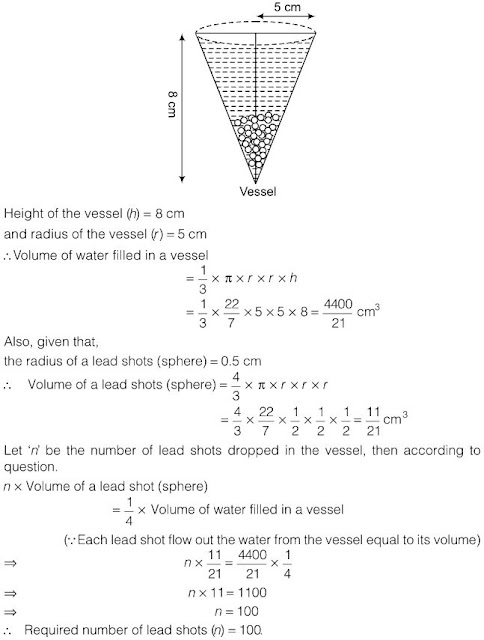6. A solid iron pole consists of a cylinder of height 220 cm and base diameter 24 cm, which is surmounted by another cylinder of height 60 cm and radius 8 cm. Find the mass of the pole, given that 1 cm3 of iron has approximately 8 g mass. (Use π = 3.14)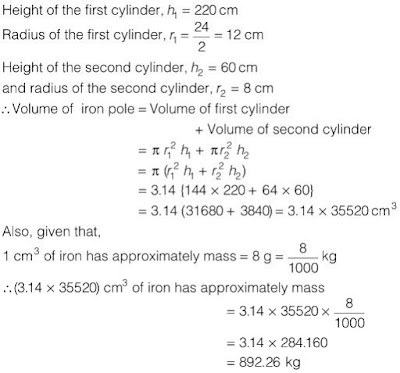7. A solid consisting of a right circular cone of height 120 cm and radius 60 cm standing on a hemisphere of radius 60 cm is placed upright in a right circular cylinder full of water such that it touches the bottom. Find the volume of water left in the cylinder, if the radius of the cylinder is 60 cm and its height is 180 cm.8. A spherical glass vessel has a cylindrical neck 8 cm long, 2 cm in diameter; the diameter of the spherical part is 8.5 cm. By measuring the amount of water it holds, a child finds its volume to be
345 cm3. Check whether she is correct, taking the above as the inside measurements and π = 3.14.

She is not correct.Exercise 13.3

1. A metallic sphere of radius 4.2 cm is melted and recast into the shape of a cylinder of radius 6 cm. Find the height of the cylinder.

Given, the radius of the sphere (r) = 4.2 cm
Radius of the cylinder (r1) = 6 cm2. Metallic spheres of radii 6 cm, 8 cm and 10 cm, respectively, are melted to form a single solid sphere. Find the radius of the resulting sphere.

Let r1, r2 and r3 be the radius of given three spheres and R be the radius of a single solid sphere.
Given, r1 = 6 cm , r2 = 8 cm and r3 =10 cm3. A 20 m deep well with diameter 7 m is dug and the earth from digging is evenly spread out to form a platform 22 m by 14 m. Find the height of the platform.

Given, the height of deep well which form a cylinder (h1) = 20 m4. A well of diameter 3 m is dug 14 m deep. The earth taken out of it has been spread evenly all around it in the shape of a circular ring of width 4m to form an embankment. Find the height of the embankment.

Given, the height of deep well which form a cylinder (h1) = 14 m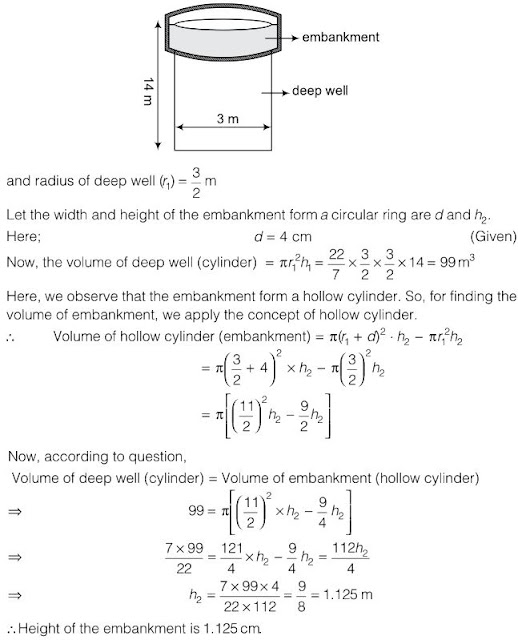5. A container shaped like a right circular cylinder having diameter 12 cm and height 15 cm is full of ice cream. The ice cream is to be filled into cones of height 12 cm and diameter 6 cm, having a hemispherical shape on the top. Find the number of such cones which can be filled with ice cream.

Let the height and radius of ice cream container (cylinder) be h1 and r1.6. How many silver coins, 1.75 cm in diameter and of thickness 2 mm, must be melted to form a cuboid of dimensions 5.5 10 3 5 . . cm cm cm  × ?

We know that, every coin has a shape of cylinder. Let radius and height of the coin are r1 and h1 respectively.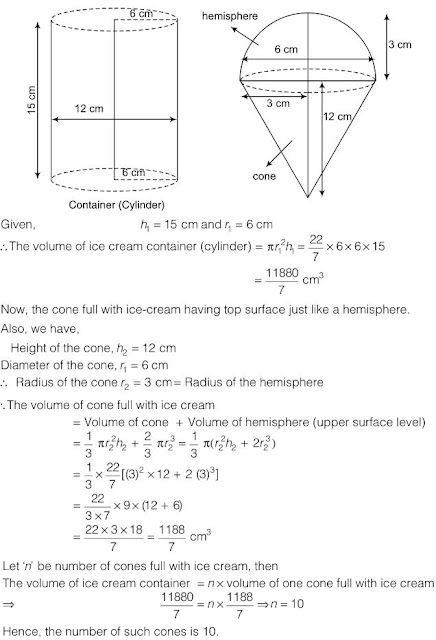7. A cylindrical bucket, 32 cm high and with radius of base 18 cm, is filled with sand. This bucket is emptied on the ground and a conical heap of sand is formed. If the height of the conical heap is 24 cm, find the radius and slant height of the heap.

Let the radius and slant height of the heap of sand are r and l.
Given, the height of the heap of sand h = 24 cm.8. Water in a canal, 6 m wide and 1.5 m deep is flowing with a speed of 10 kmh−1. How much area will it irrigate in 30 minutes, if 8 cm of standing water is needed?

Given, speed of flow of water (l) = 10 kmh-1 = 10 × 1000 mh−1
Area of canal = 6 × 1.5 = 9m29. A farmer connects a pipe of internal diameter 20 cm from a canal into a cylindrical tank in her field, which is 10 m in diameter and 2 m deep. If water flows through the pipe at the rate of 3 kmh−1, in how much time will the tank be filled?

Given, speed of flow of water = 3 kmh−1 = 3×1000 mh−1
∴ Length of water in 1 h = 3000 mExercise 13.4

1. A drinking glass is in the shape of a frustum of a cone of height 14 cm. The diameters of its two circular ends are 4 cm and 2 cm. Find the capacity of the glass.

Let the height of the frustum of a cone be h.2. The slant height of a frustum of a cone is 4 cm and the perimeters (circumference) of its circular ends are 18 cm and 6 cm. Find the curved surface area of the frustum.

Let the slant height of the frustum be l and radius of the both ends of the frustum be r1 and r2.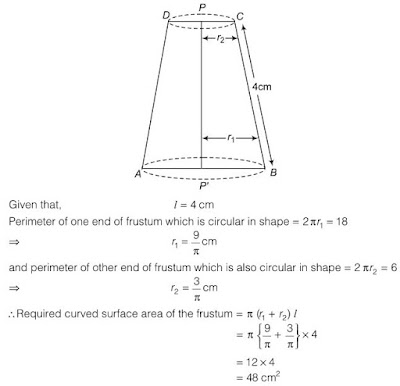3. A fez, the cap used by the turks, is shaped like the frustum of a cone (see figure). If its radius on the open side is 10 cm, radius at the upper base is 4 cm and its slant height is 15 cm, find the area of material used for making it.Let the slant height of fez be l and the radius of upper end which is closed be r1 and the other end which is open be r2.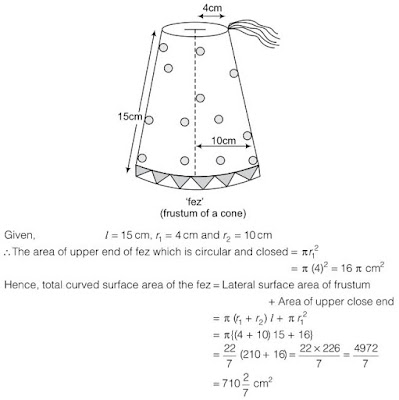4. A container, opened from the top and made up of a metal sheet, is in the form of a frustum of a cone of height 16 cm with radii of its lower and upper ends as 8 cm and 20 cm, respectively. Find the cost of the milk which can completely fill the container, at the rate of ` 20 per litre. Also find the cost of metal sheet used to make the container. If it costs ₹8 per 100 cm2. (Take π = 3.14)

Let h be the height of the container, which is in the form of a frustum of a cone whose lower end is closed and upper end is opened. Also, let the radius of its lower end be r1 and upper end be r2.5. A metallic right circular cone 20 cm high and whose vertical angle is 60º is cut into two parts at the middle of its height by a plane parallel to its base. If the frustum so obtained be drawn into a wire of diameter 1/16 cm, find the length of the wire.

Let r1 and r2 be the radii of the frustum of upper and lower ends cut by a plane. Given, height of the cone = 20 cm.
∴ Height of the frustum = 10 cm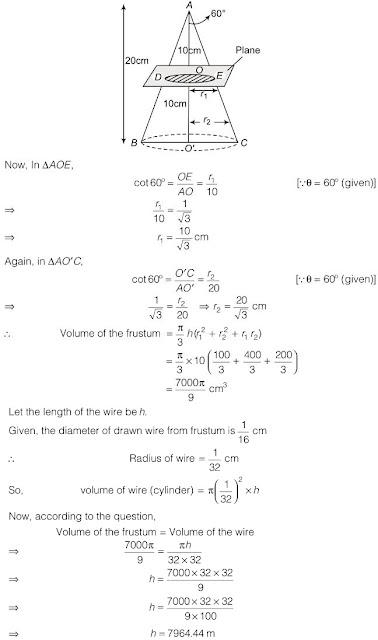Exercise 13.5 (Optional)

1. A copper wire, 3 mm in diameter, is wound about a cylinder whose length is 12 cm and diameter 10 cm, so as to cover the curved surface of the cylinder. Find the length and mass of the wire, assuming the density of copper to be 8.88 g per cm3.

Since, the diameter of the wire is 3 mm.
When a wire is one round wound about a cylinder, it covers a 3 mm of length of the cylinder.
Given, length of the cylinder = 12 cm = 120 mm
∴ Number of rounds to cover 120 mm = 120/3 = 40
Given, diameter of a cylinder is d = 10 cm
∴ Radius, r = 10/2 = 5 cm
∴ Length of wire required to complete one round = 2πr = 2π(5) = 10π cm
∴ Length of the wire in covering the whole surface
= Length of the wire in completing 40 rounds
= 10π × 40 = 400π cm
= 400 × 3.14 = 1256 cm
Now, radius of copper wire = 3/2 mm = 3/20 cm2. A right triangle, whose sides are 3 cm and 4 cm (other than hypotenuse) is made to revolve about its hypotenuse. Find the volume and surface area of the double cone so formed. (Choose value of π as found appropriate).

Here, ABC is a right angled triangle at A and BC is the hypotenuse.3. A cistern, internally measuring 150 cm × 120 cm× 110 cm, has 129600 cm3 of water in it. Porous bricks are placed in the water until the cistern is full to the brim. Each brick absorbs one-seventeenth of its own volume of water. how many bricks can be put in without overflowing the water, each brick being 22.5 cm × 7.5 cm × 6.5 cm?

Given, internally dimensions of cistern = 150 cm×120 cm×110 cm
∴ Volume of cistern = 150×120×110
= 1980000 cm3
Volume of water = 129600 cm3
∴ Volume of cistern to be filled = 1980000 - 129600 = 1850400 cm3
Let required number of bricks = n4. In one fortnight of a given month, there was a rainfall of 10 cm in a river valley. If the area of the valley is 97280 km2, show that the total rainfall was approximately equivalent to the addition to the normal water of three rivers each 1072 km long, 75 m wide and 3 m deep.

Given, area of the valley = 97280 km2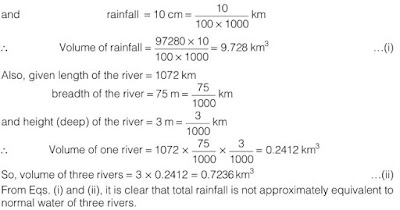5. An oil funnel made of tin sheet consists of a 10 cm long cylindrical portion attached to a frustum of a cone. If the total height is 22 cm, diameter of the cylindrical portion is 8 cm and the diameter of the top of the funnel is 18 cm, find the area of the tin sheet required to make the funnel.Given, oil funnel is a combination of a cylinder and a frustum of a cone.
Also, given height of cylindrical portion h = 10 cm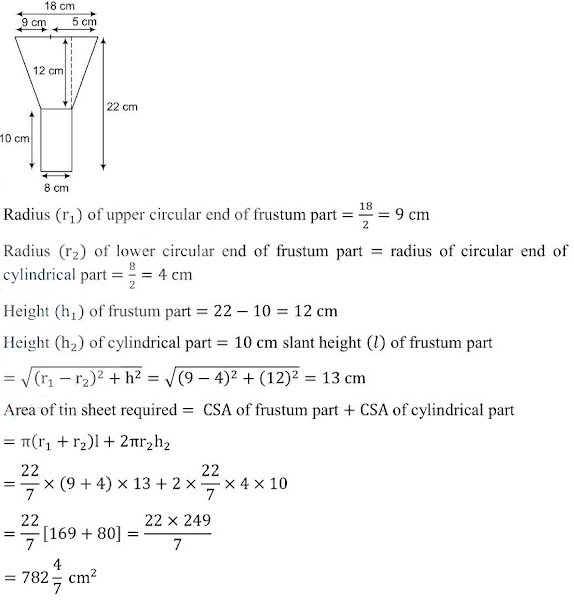6. Derive the formula for the curved surface area and total surface area of the frustum of a cone. Using the symbols as explained.

Leth be the height, l be the slant height and r1 and r2 be the radii of the bases (r1>r2) of the frustum of a cone. We complete the conical part OCD.The frustum of the right circular cone can be viewed as the difference of the two right circular cones OAB and OCD. Let slant height of the cone OAB be l1 and its height be h1 i.e.,OB = OA = l1 and OP = h1
The frustum of the right circular cone can be viewed as the difference of the two right circular cones OAB and OCD. Let slant height of the cone OAB be l1 and its height be h1 i.e., OB = OA = l1 and OP = h1
Then in ∆ACE,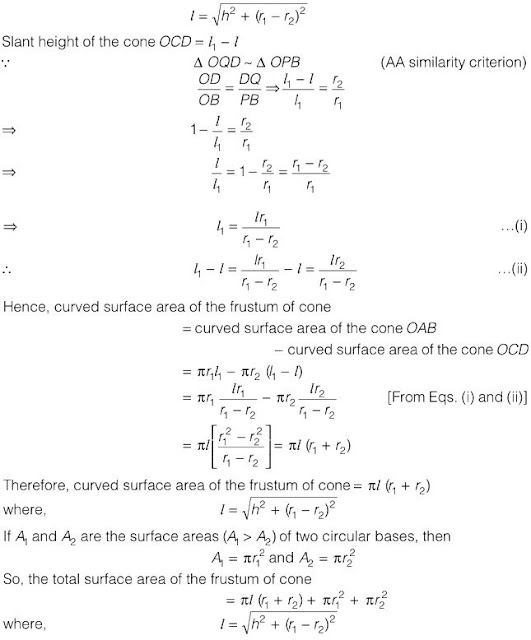7. Derive the formula for the volume of the frustum of a cone given to you in the section 13.5 using the symbols as explained.